# Memory DPD

NI-RFmx SpecAn 3.0 Help

Edition Date: January 2019

Part Number: 374264L-01

»View Product InfoDownload Help (Windows Only)

In addition to being nonlinear devices, the instantaneous output of a power amplifiers is a function not only of the current signal amplitude and phase but also of the past signal amplitude and phase. This is commonly referred to as memory in a device. The following equation expresses the instantaneous output.The most general form that the nonlinear function can take is the Volterra Series. The following equation is a discrete version of Volterra Series .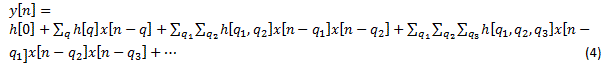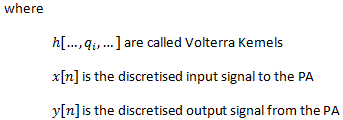To digitally compensate for memory and nonlinearity of the PAs, the most general structure of DPD also takes the same form as equation (4). While Volterra Series is the most general way of modeling DPD, extraction of large number of Volterra Kernels is usually both computationally intensive and unnecessary. Instead, many pruned versions of Volterra Series have been developed. DPD measurement in RFmx supports two such DPD models–Memory Polynomial Model and Generalized Memory Polynomial Model.

## Memory Polynomial Model

PAs that display significant memory effects are not satisfactorily linearized using the LUT approach of DPD. Such PAs require compensation for memory effects. A well known structure for memory and nonlinearity compensation in PAs is the memory polynomial model , the complex baseband representation of which is shown in the following equation.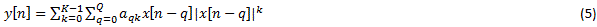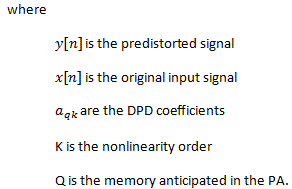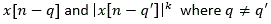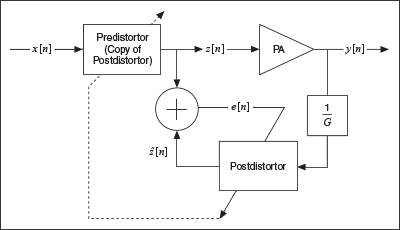## Generalized Memory Polynomial (GMP)

PAs that display significantly high memory effects are not completely linearized using memory polynomial. GMP  can be employed for such PAs. For the purpose of further discussion on GMP, we shall introduce the following terms:

 Expression Type Description x[n] Signal Signal value at the current time index x[n-q] Signal Signal value q samples before the current time index |x[n]|k Envelope Signal's kth order envelope of the signal x[n] |x[n-q]|k Envelope Signal's kth order envelope of the signal x[n-q] |x[n-q-m]|k Envelope Signal's kth order envelope lagging behind the signal x[n-q] by m samples |x[n-q+m]|k Envelope Signal's kth order envelope leading the signal x[n-q] by m samples

The following equation is a complex baseband representation of the GMP-based predistorter.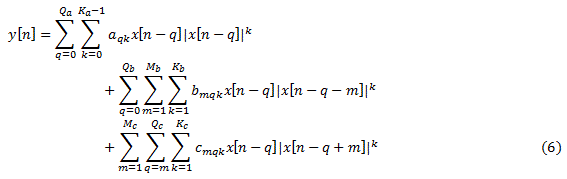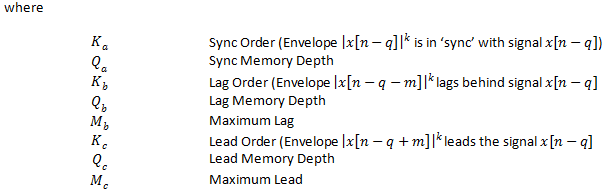The first double sum in GMP in equation (6) is exactly the same as MPM, capturing the memory effects and nonlinearity of the PA. The other two triple sums account for the lag and lead between the signal and envelope, compensating for the staggered memory effects of the cross terms.

# References

 Schetzen, Martin. "The Volterra and Wiener theories of nonlinear systems." (1980).
 Ding, Lei, et al. "Memory polynomial predistorter based on the indirect learning architecture." Global Telecommunications Conference, 2002. GLOBECOM'02. IEEE. Vol. 1. IEEE, 2002.
 Morgan, Dennis R., et al. "A generalized memory polynomial model for digital predistortion of RF power amplifiers." Signal Processing, IEEE Transactions on54.10 (2006): 3852-3860.